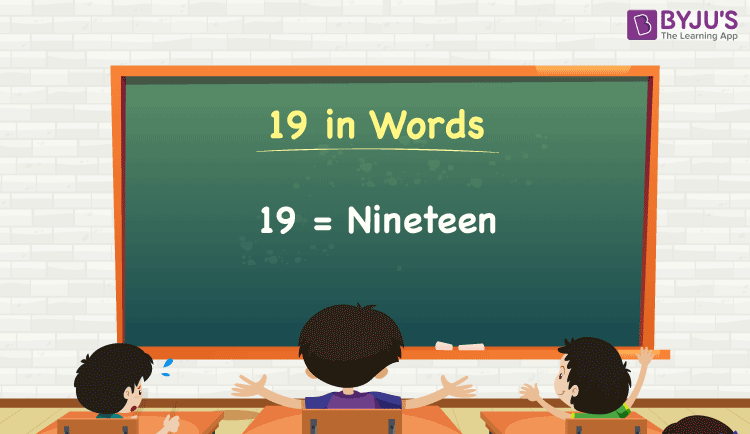# 19 in Words

19 in words is written as Nineteen. Using a place value chart, we can derive the number 19 in words. If you have 19 pens, you can say, “I have nineteen pens with me”. 19 is a cardinal number since it denotes some specific quantity.

 19 in words: Nineteen Nineteen in numerical form: 19

19 in English Words

We generally write numbers in words using the English alphabet. Thus, we can read 19 using the words in English as “Nineteen”.## How to Write 19 in Words?

19 is a two-digit number so let’s make a place value chart with two columns as shown below.

 Tens Ones 1 9

Here, the digit at the tens place is 1 and the units place is 9. So, let us expand these digits as per the place values and combine the results to get the final number.

1 × Tens + 9 × Ones

= 1 × 10 + 9 × 1

= 10 + 9

= 19

= Nineteen

Therefore, 19 in words = Nineteen.

Interesting way of writing 19 in words:

1 = One

9 = Nine

10 = Ten

10 + 9 = 19

Ten + Nine = Nineteen

### Facts About the Number 19

19 is a natural number that is the successor of 18 and the predecessor of 20.

19 in words – Nineteen

Is 19 an odd number? – Yes

Is 19 an even number? – No

Is 19 a prime number? – Yes

Is 19 a composite number? – No

Is 19 a perfect square number? – No

Is 19 a perfect cube number? – No

### 1 to 20 in Words

 1 in words = One 11 in words = Eleven 2 in words = Two 12 in words = Twelve 3 in words = Three 13 in words = Thirteen 4 in words = Four 14 in words = Fourteen 5 in words = Five 15 in words = Fifteen 6 in words = Six 16 in words = Sixteen 7 in words = Seven 17 in words = Seventeen 8 in words = Eight 18 in words = Eighteen 9 in words = Nine 19 in words = Nineteen 10 in words = Ten 20 in words = Twenty

## Frequently Asked Questions on 19 in Words

Q1

### How do you write 19 in English words?

The spelling of 19 in English words is Nineteen, which means 19 in words can be expressed as nineteen.

Q2

### Write the value of 13 + 6 in words.

13 + 6 = 19
Thirteen + Six = Nineteen
Therefore, 13 + 6 is equal to nineteen.

Q3

### How do you write Rs. 19 in words?

We can write Rs. 19 in words as Nineteen rupees.